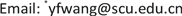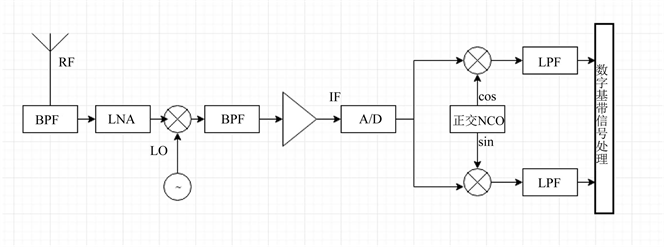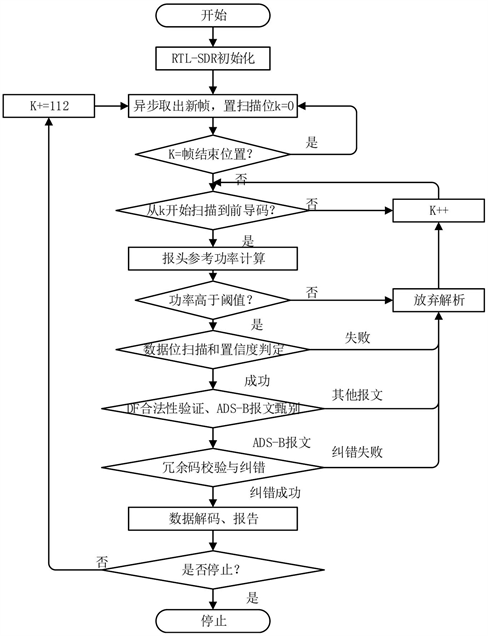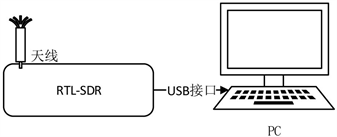﻿ 基于RTL-SDR的ADS-B应答信号处理平台的研究与实现 The Research and Implement of ADS-B Response Signal Processing Platform Based on RTL-SDR

Journal of Image and Signal Processing
Vol. 08  No. 02 ( 2019 ), Article ID: 29441 , 7 pages
10.12677/JISP.2019.82005

The Research and Implement of ADS-B Response Signal Processing Platform Based on RTL-SDR

Jincheng Yan, Yunfeng Wang*

Department of Computer Science and Technology, Sichuan University, Chengdu SichuanReceived: Mar. 6th, 2019; accepted: Mar. 17th, 2019; published: Mar. 27th, 2019ABSTRACT

By studying on ADS-B (Automatic dependent surveillance-broadcast) response signal based on the 1090ES data link, this paper implements a ADS-B response signal processing platform with C++ language in Windows environment under the device supports of RTL-SDR and PC. The platform can successfully receive and parse ADS-B response signals, which proves the possibility with software radio ways under low cost.2. RTL-SDR技术介绍

RTL-SDR是一种低成本的小型软件无线电处理平台，由R820T调谐器和R2832U芯片组成。它可接收25 MHz至1.75 GHz范围内的射频信号，支持的最大采样率为2.8 MHz。通过模拟电路，RTL-SDR把射频信号降到中频，然后经过模数转换，用数字信号处理的方式将中频信号搬到基带，这实际上是一种超外差式数字中频接收机   。为进一步降低后续软件部分对信号的处理压力，减少处理信号量，RTL-SDR对基带信号进行数字抽取，使其采样频率降低在2.8 MHz以下，从USB输出8位基带IQ信号，对于该基带信号，可以使用编写软件的方法在PC中对其进行处理，RTL-SDR的结构如图1Figure 1. The structure of RTL-SDR

RTL-SDR输出的IQ两路信号共16 bit，即一个样本点为2B。利用开源的RTL-SDR驱动提供的API初始化RTL-SDR设备，设定采样率为2 MHz，数据缓存块大小为256 KB，则输入一个完整的数据块需要64 ms，采用异步读取的方式，使程序在64 ms内完成对数据块的处理，可保证信号处理的实时性，具体的程序设计如图2Figure 2. Design of ADS-B response signal processing program based on RTL-SDR

3.1. 前导码检测

${S}_{i}=\sqrt{{I}_{i}^{2}+{Q}_{i}^{2}}$ (1)

3.1.1. 前导码脉冲位置检测及开始位置确定

$\begin{array}{l}{S}_{k}>{S}_{k+1},\\ {S}_{k+1}<{S}_{k+2},\\ {S}_{k+2}>{S}_{k+3},\\ {S}_{k+3}<{S}_{k+4},\\ {S}_{k+6}<{S}_{k+7},\\ {S}_{k+7}>{S}_{k+8},\\ {S}_{k+8}<{S}_{k+9},\\ {S}_{k+9}>{S}_{k+10}\end{array}$ (2)

$\begin{array}{l}\stackrel{¯}{S}=\left({S}_{k}+{S}_{k+2}+{S}_{k+7}+{S}_{k+9}\right)/4\\ {S}_{k+i}<\frac{\stackrel{¯}{S}}{3},i=4,5,11,12,13,14\end{array}$ (3)

3.1.2. 参考功率计算

3.2. 数据块扫描

3.2.1. 数据位和置信度判定

${C}_{i}=\left\{\begin{array}{l}1,{S}_{k+16+2×i}\ge {S}_{k+16+2×i+1}\\ 0,{S}_{k+16+2×i}<{S}_{k+16+2×i+1}\end{array}$ (4)

${B}_{i}=\left\{\begin{array}{l}1,{S}_{k+16+2×i}\ne {S}_{k+16+2×i+1}\\ 0,{S}_{k+16+2×i}={S}_{k+16+2×i+1}\end{array}$ (5)3.3. 循环冗余校验

S模式采用24位循环冗余校验码(Cyclic Redundancy Check, CRC)进行检错，本身不提供纠错功能，但是基于置信度，CRC拥有一定的纠错功能。采用蛮力纠错技术和保守纠错技术结合的方式可以最多纠错12位数据，但此时要求出错数据的窗口大小不超过24  。

3.4. 解码

4. 平台实现与处理报告分析Figure 3. The structure of receive platform

5. 总结

The Research and Implement of ADS-B Response Signal Processing Platform Based on RTL-SDR[J]. 图像与信号处理, 2019, 08(02): 29-35. https://doi.org/10.12677/JISP.2019.82005

1. 1. 吴凯峰, 宋东, 刘柏兵, 等. 飞机自动相关监视信号接收系统设计[J]. 传感器与微系统, 2018(9): 105.

2. 2. 李耀. S模式ADS-B接收机解码板的研究及实现[D]: [硕士学位论文]. 成都: 电子科技大学, 2008.

3. 3. 陈士毅. 模式S应答处理中的数据处理[D]: [硕士学位论文]. 成都: 电子科技大学, 2003.

4. 4. 王伟玮, 涂榫. 基于ADS-B和RTL-SDR的空中交通监视系统[J]. 微型机与应用, 2016, 35(17): 80-83.

5. 5. Stewart, R.W. (2015) Software Defined Radio Using MATLAB & Simulink and the RTL-SDR. Department of Electronic and Electrical Engineering University of Strathclyde Glasgow, Scotland.

6. 6. 陈祝明. 软件无线电技术基础[M]. 北京: 高等教育出版社, 2007.

7. 7. 吴杰, 郭建华, 蒋凯, 等. ADS-B二重交织信号时域分离算法[J]. 通信技术, 2017(10): 2184.

8. 8. 张涛, 唐小明, 宋洪良. 一种ADS-B报头互相关检测方法[J]. 电讯技术, 2016(2): 156.

9. 9. 郑植, 练马林. 模式S应答处理中报头检测算法的研究与实现[J]. 电子科技大学学报, 2008, 37(1): 66-70.

10. 10. 王菲. 基于1090MHz ES数据链ADS-B关键技术研究[D]: [硕士学位论文]. 成都: 电子科技大学, 2009.

11. 11. 张尉. 空管二次雷达[M]. 北京: 国防工业出版社, 2017.

12. 12. 李明洋, 时宏伟. 二次雷达S模式应答信号与ADS-B信号的甄别研究[J]. 微型机与应用, 2015, 34(20): 67-69.

13. 13. 郭焘, 田忠. ADS-B系统接收处理的关键算法研究与实现[J]. 火控雷达技术, 2013, 42(3): 43-51.

14. 14. 张鹏, 何光亮. 1090ES关键信息解码算法优化及实现[J]. 现代电子技术, 2018(3): 30.

15. 15. 王尔申, 翟秋刚, 李玉峰, 等. 应用Qt的ADS-B报文解析研究与实现[J]. 电光与控制, 2018(4): 69.

16. NOTES

*通讯作者。